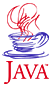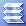Home : Course Map : Chapter 4 : Java : Tech : Random Numbers in JavaIntroduction Inheritance   Demo 1 Overriding   Demo 2a   Demo 2b this,super MoreConstructors   Demo 3 Abstraction Interface    Demo 4 Casting References MoreAboutArrays Object class Class Summary Exercises
 Supplements Override/Overload Annotation-J2SE5.0 Java Security Class Loading Class Verifier SecurityManager
 About JavaTech      Codes List      Exercises      Feedback      References      Resources      Tips      Topic Index      Course Guide      What's New

Random values can be obtained from the Math class using:

public static double random()

which produces pseudo-random double values in the range

0.0 <= r < 1.0

The first time it is invoked, it initializes with the current time.

The java.util.Random class provide a more extensive set of random generators. Two constructors Random() and Random(long seed) offer the options of initialization from the current time or from a specific seed value.

The methods in the Random class include:

• nextInt() - integers in the range 0 <= r < 2**32
• nextInt(int n) - integers in the range 0 <= r < n (available with Java 1.2)
• nextBoolean(int n) - randomly chosen true/false (available with Java 1.2)
• nextGaussian() - random double values with mean 0.0 and sigma of 1.0. (available with Java 1.2)

Random Number Algorithm

The Random class uses a linear congruential algorithm (descriptions in Knuth and Press) with a 48-bit seed. If the constructor Random(long) or the setSeed(long) method is invoked, the algorithm will use only the lower 48 bits of the seed value.

Random number generator formulas actually produce a sequence of numbers that eventually repeat. For the same seed value a formula will produce the same sequence. A seed simply selects where in the sequence to start. The generator will eventually repeat that seed value and start the same sequence again. Compared to the randomness of physical fluctuations, such as in radio noise, these formulas are said to produce pseudo-random numbers.

To insure that applications ported to different platforms give the same results, all implementations of Java must use the same algorithm so that the same seed returns the same sequence regardless of the platform.

The linear congruential formula in Java goes as

xi+1 = (a * xi + c) mod m

As discussed in Knuth and Press, you should use such formulas with care. They can produce random number sequences of a length up to m but not necessarily that long. The length depends on the set of a, c, and m values chosen.

Also, if you grab consecutive sequences of numbers of K length, and plot them as points in K dimensional space, they will not fully populate the volume randomly but instead lie on K-1 dimnsional planes. There will be no more than m1/k planes and possible less. If you need to create points in a space this way, you should shuffle the values obtained from the generator. (See Press for suggested shuffle algorithms.)

In Java the values in the linear congruential formula are

a = 0x5DEECE66DL
c = 11
m = 248 - 1.

The actual code in next(int bits) goes as

synchronized protected int next(int bits) {
seed = (seed * 0x5DEECE66DL + 0xBL) & ((1L << 48) - 1);
return (int)(seed >>> (48 - bits));
}

Here the mod operation comes via the AND operation since m in this case has all 47 bits set to one.

This method is protected (see Chapter 5: Java : Access Rules). The public random number methods accessible by all classes invoke this method. For example, nextInt() simply includes the statement

return next(32);

The nextLong() method invokes next(32) shifts the result by 32 bits to the left, invokes next(32) again and then OR's the two values together to obtain a 64 bit random number:

((long)next(32) << 32) + next(32);

The nextFloat() method provides 224 values in the range range 0.0f (inclusive) to 1.0f (exclusive). The next(int bits) method provides a value up to 24 bits long and then this is divided by 224.

next(24) / ((float)(1 << 24));

The nextDouble() method provdides 253 values in the range 0.0d (inclusive) to 1.0d (exclusive) using the statement

(((long)next(26) << 27) + next(27)) / (double)(1L << 53)

The nextBoolean() method uses the statement

return next(1) != 0;

See the java.util.Random class specification for more detailed descriptions for each of the next{type} methods.

Note: we will discuss the Gaussian (or "normally") distributed generation algorithm used for the nextGaussian() method in Chapter 6 : Tech : Non-uniform Distributions.

References & Web Resources

 Tech MoreComplexClass ImprovedHistogram JavaRandomNums Vectors & Matrices Exercises
 Physics Runge-Kutta 2nd   Demo 1 Runge-Kutta 4th   Demo 2 BoundaryVal.Prob Shooting Method   Demo 3 ExercisesPart I Part II Part III Java Core 1  2  3  4  5  6  7  8  9  10  11  12 13 14 15 16 17 18 19 20 21 22 23 24 Supplements 1  2  3  4  5  6  7  8  9  10  11  12 Tech 1  2  3  4  5  6  7  8  9  10  11  12 Physics 1  2  3  4  5  6  7  8  9  10  11  12

Java is a trademark of Sun Microsystems, Inc.